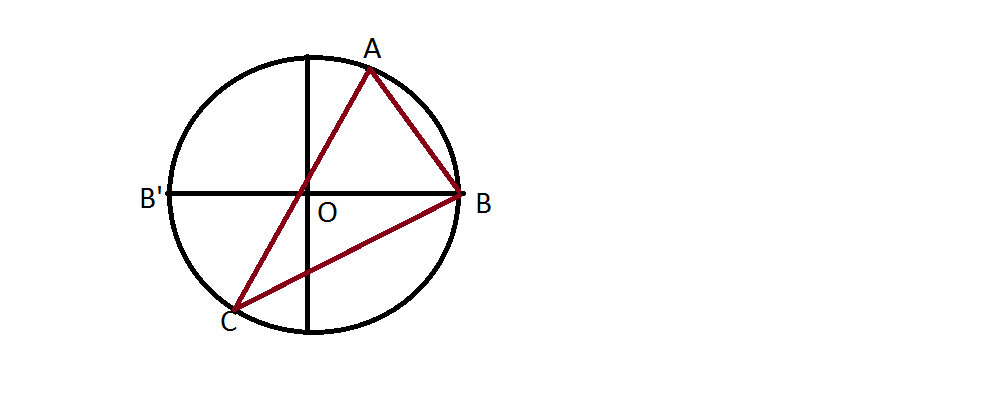# Probability

When any three points are selected from a circle, what is the probability that they will form an obtuse-angled triangle?

•Shaswata Roy ·Let ABC be a triangle , O the center of circumcircle and BB' a diameter.

Let's try and find out the probability that it is an acute angled triangle.(Since it involves less complications)

If ABC is acute angled then O will be included inside ΔABC.

(Using normal coordinate system with center as origin)For ΔABC to be acute A should lie in 2nd quadrant and C should lie in 3rd quadrant.

Hence probability that ΔABC is acute = 1/2*/12=1/4

Therefore probability that the triangle is obtuse = 3/4